Sara Stoudt true
07-10-2018

# Week 15

Craft Beer USA

data.world

thrillist.com

``````require(readxl)
require(dplyr)
require(ggplot2)
require(stringr)
``````
``````setwd("~/Desktop/tidytuesday/data/2018/2018-07-10")
beers <- read_excel("week15_beers.xlsx", sheet = 1)
brewer <- read_excel("week15_beers.xlsx", sheet = 2)
beer <- inner_join(beers, brewer, by = c("brewery_id" = "id"))
``````
``````byState <- beer %>%
group_by(state) %>%
summarise(numBrewer = length(unique(brewery_id)), count = n(), mabv = mean(abv, na.rm = T))
counties <- map_data("county")
state <- map_data("state")

stateInfo <- cbind.data.frame(abb = state.abb, name = tolower(state.name))

state <- inner_join(state, stateInfo, by = c("region" = "name"))

all_state <- inner_join(state, byState, by = c("abb" = "state"))
``````

This palette isn’t very visually appealing, but in the spirit of beer, I’ll use it anyway.

``````# https://www.reddit.com/r/beer/comments/4gd24e/the_hex_colour_palette_of_beer/
beerPal <- c("#F3F993", "#F5F75C", "#F6F513", "#EAE615", "#E0D01B", "#D5BC26", "#CDAA37", "#C1963C", "#BE8C3A", "#BE823A", "#C17A37", "#BF7138", "#BC6733", "#B26033", "#A85839", "#985336", "#8D4C32", "#7C452D", "#6B3A1E", "#5D341A", "#4E2A0C", "#4A2727", "#361F1B", "#261716", "#231716", "#19100F", "#16100F", "#120D0C", "#100B0A", "#050B0A")
``````

## Where to Bar Crawl?

``````ggplot(data = state, mapping = aes(x = long, y = lat, group = group)) +
geom_polygon(data = all_state, aes(fill = mabv), color = "grey") +
labs(fill = "mabv") +
theme_void() +
geom_path(data = state, aes(x = long, y = lat, group = group), color = "black") +
ggtitle("Where is the beer strongest?")
``````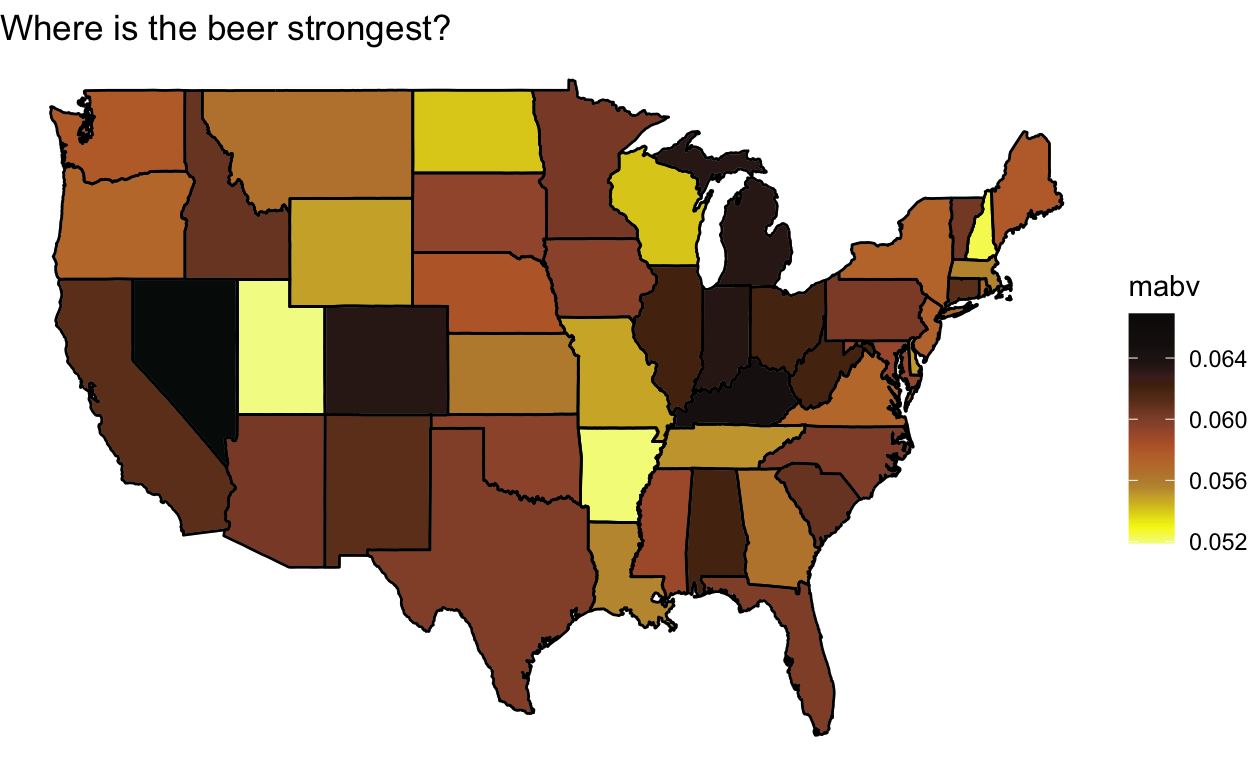A stark (and believable) difference between Nevada and Utah.

``````ggplot(data = state, mapping = aes(x = long, y = lat, group = group)) +
geom_polygon(data = all_state, aes(fill = numBrewer), color = "grey") +
labs(fill = "numBrewer") +
theme_void() +
geom_path(data = state, aes(x = long, y = lat, group = group), color = "black") +
ggtitle("Where are the most breweries?")
``````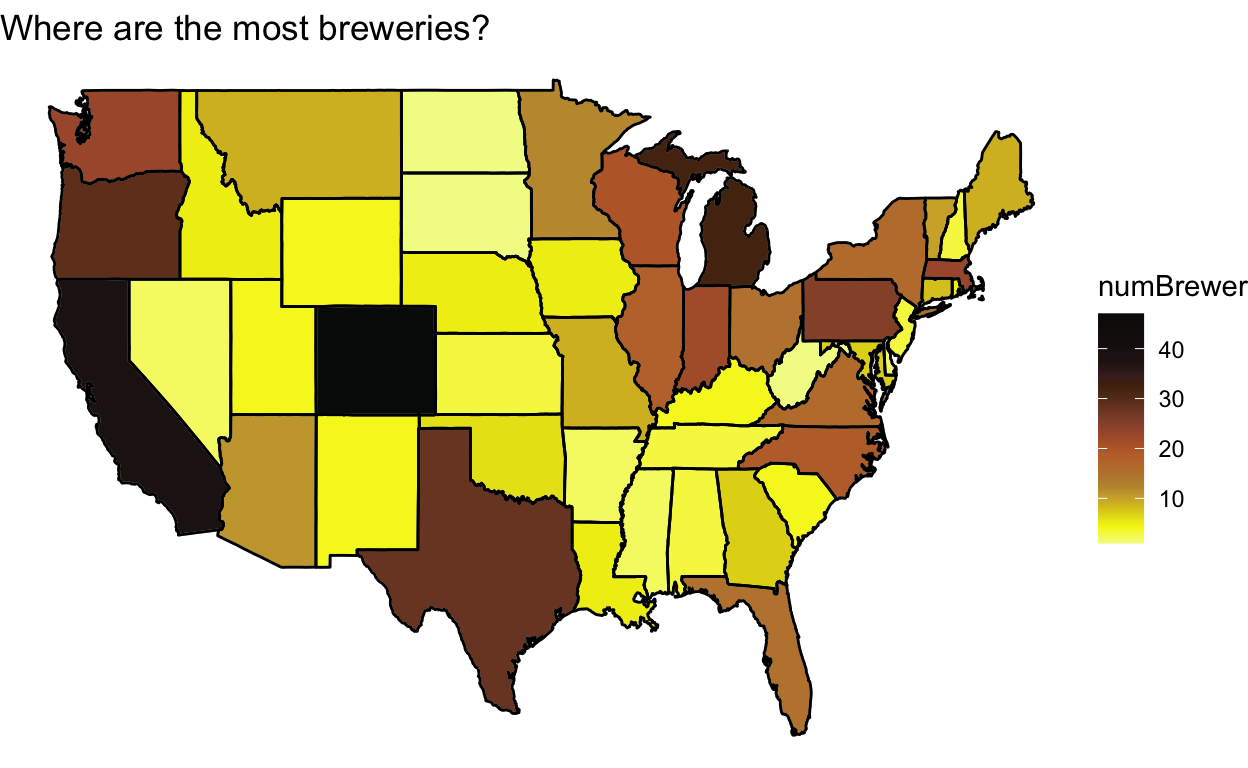``````ggplot(data = state, mapping = aes(x = long, y = lat, group = group)) +
geom_polygon(data = all_state, aes(fill = count / numBrewer), color = "grey") +
labs(fill = "beerPerBrewer") +
theme_void() +
geom_path(data = state, aes(x = long, y = lat, group = group), color = "black") +
ggtitle("Where is the largest variety?")
``````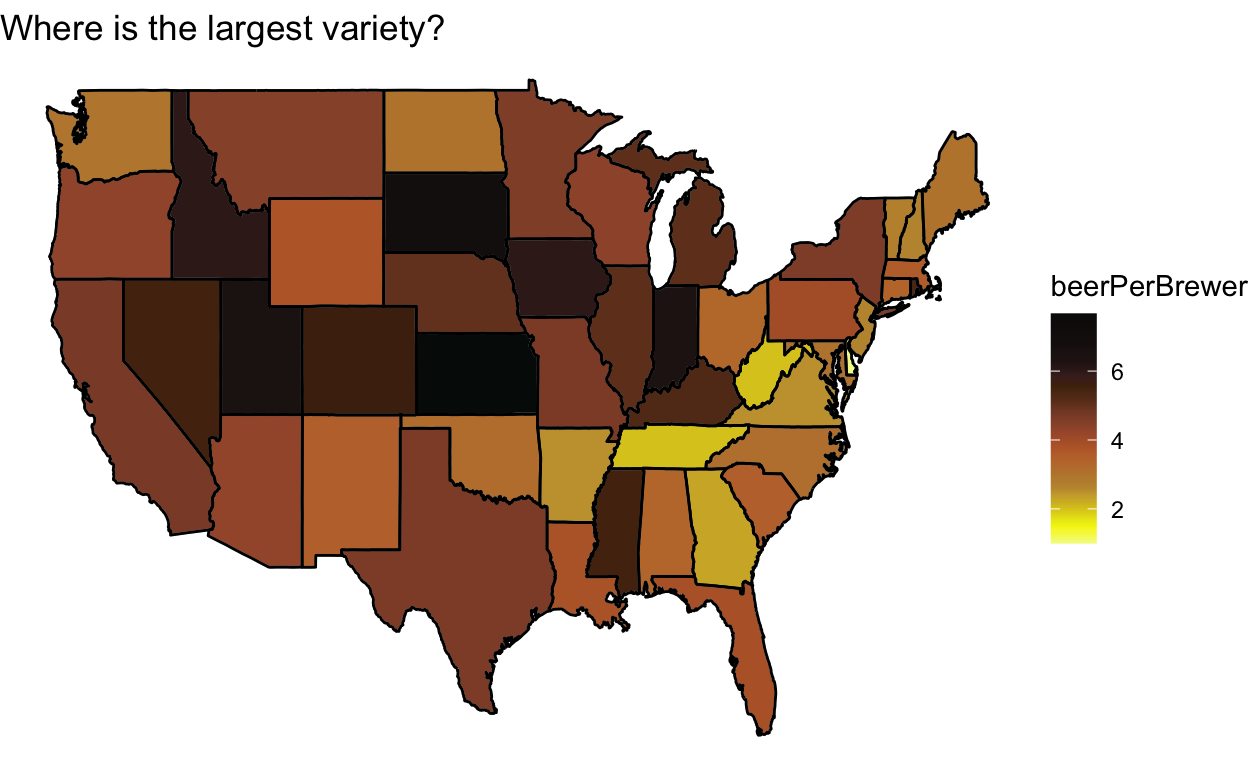Surprisingly Kansas is where it is at!

``````beer %>%
group_by(city, state) %>%
summarise(count = n(), numBrewer = length(unique(brewery_id))) %>%
arrange(desc(count))
``````
``````# A tibble: 401 x 4
# Groups:   city 
city         state count numBrewer
<chr>        <chr> <int>     <int>
1 Grand Rapids MI       66         3
2 Chicago      IL       55         9
3 Portland     OR       52        11
4 Indianapolis IN       43         4
5 San Diego    CA       42         8
6 Boulder      CO       41         9
7 Denver       CO       40         8
8 Brooklyn     NY       38         4
9 Seattle      WA       35         9
10 Longmont     CO       33         1
# … with 391 more rows``````
``````beer %>%
group_by(city, state) %>%
summarise(count = n(), numBrewer = length(unique(brewery_id))) %>%
arrange(desc(numBrewer))
``````
``````# A tibble: 401 x 4
# Groups:   city 
city          state count numBrewer
<chr>         <chr> <int>     <int>
1 Portland      OR       52        11
2 Boulder       CO       41         9
3 Chicago       IL       55         9
4 Seattle       WA       35         9
5 Austin        TX       25         8
6 Denver        CO       40         8
7 San Diego     CA       42         8
8 Bend          OR       11         6
9 Portland      ME       12         6
10 San Francisco CA       32         5
# … with 391 more rows``````

Somebody please tell me about the hidden gem of Grand Rapids. Apparently, it is Beer City, USA.

## Styles

There are too many styles, so I pick some major ones and investigate them.

``````stout <- beer[str_detect(beer\$style, "Stout"), ]
american <- beer[str_detect(beer\$style, "American"), ]
ipa <- beer[str_detect(beer\$style, "IPA"), ]
``````

True American?

``````byStateA <- american %>%
group_by(state) %>%
summarise(numBrewer = length(unique(brewery_id)), count = n(), mabv = mean(abv, na.rm = T))

all_state <- inner_join(state, byStateA, by = c("abb" = "state"))

ggplot(data = state, mapping = aes(x = long, y = lat, group = group)) +
geom_polygon(data = all_state, aes(fill = numBrewer), color = "grey") +
labs(fill = "numBrewer") +
theme_void() +
geom_path(data = state, aes(x = long, y = lat, group = group), color = "black")
``````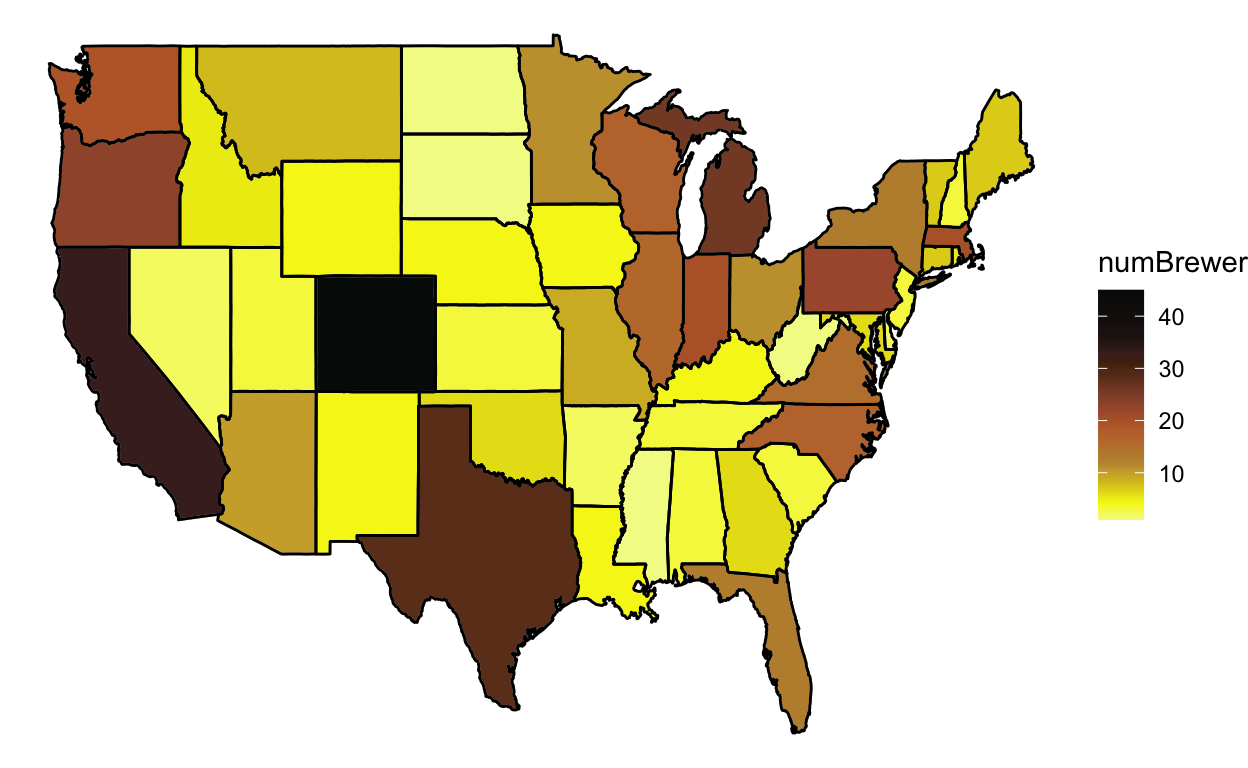``````ggplot(data = state, mapping = aes(x = long, y = lat, group = group)) +
geom_polygon(data = all_state, aes(fill = count / numBrewer), color = "grey") +
labs(fill = "beerPerBrewer") +
theme_void() +
geom_path(data = state, aes(x = long, y = lat, group = group), color = "black")
``````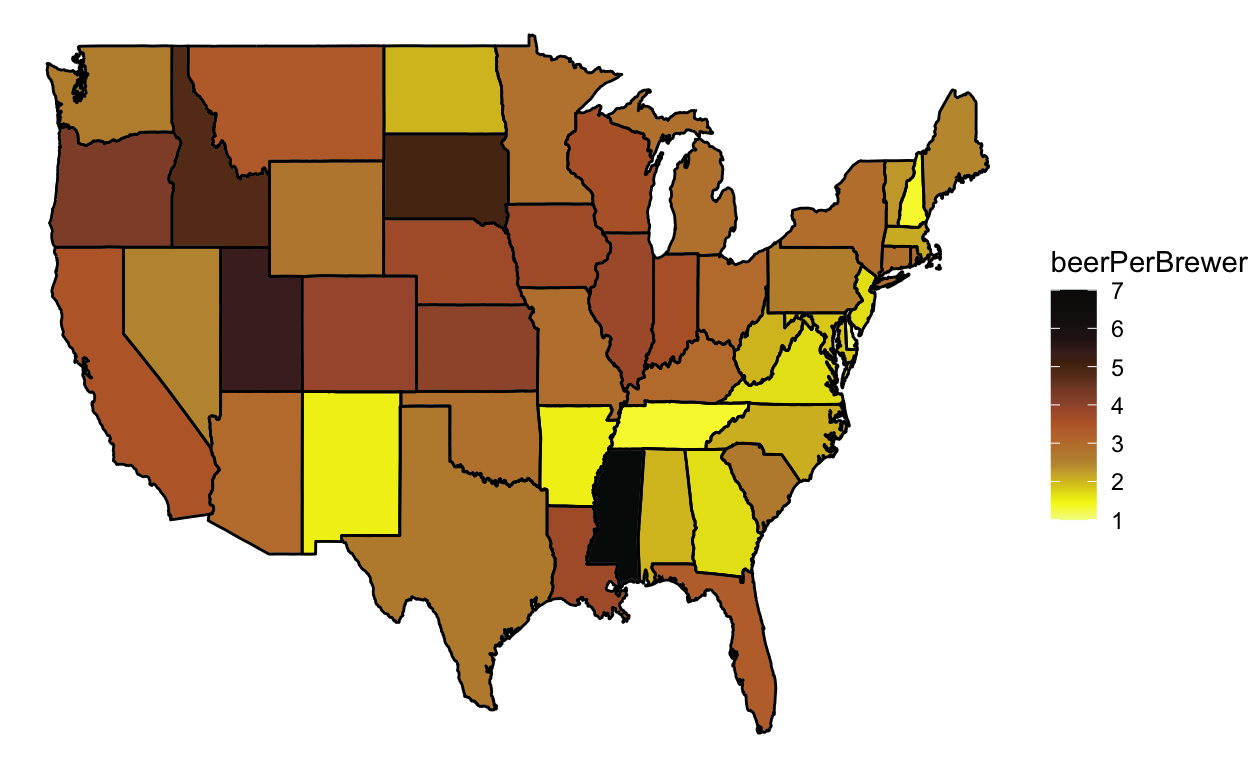Mississippi: American Beer’s Hearland

Representing my namesake

``````byStateS <- stout %>%
group_by(state) %>%
summarise(numBrewer = length(unique(brewery_id)), count = n(), mabv = mean(abv, na.rm = T))

all_state <- inner_join(state, byStateS, by = c("abb" = "state"))

ggplot(data = state, mapping = aes(x = long, y = lat, group = group)) +
geom_polygon(data = all_state, aes(fill = numBrewer), color = "grey") +
labs(fill = "numBrewer") +
theme_void() +
geom_path(data = state, aes(x = long, y = lat, group = group), color = "black")
``````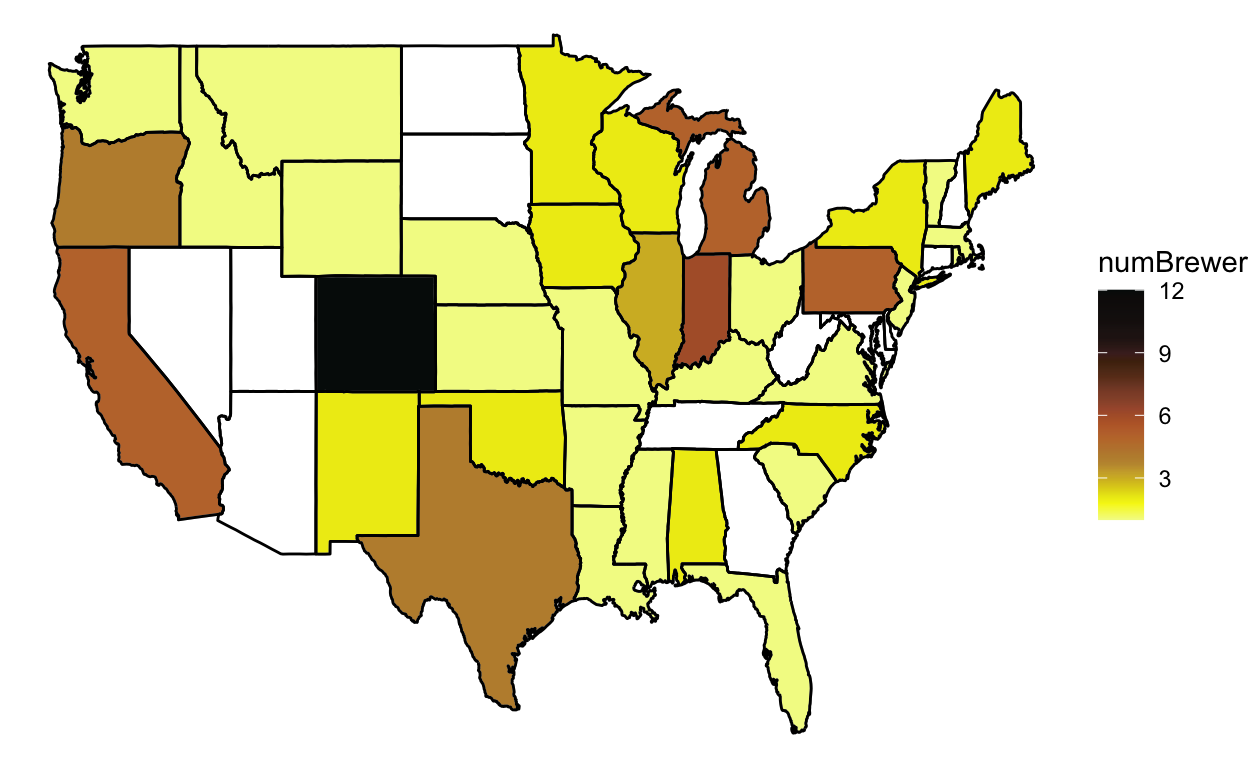``````ggplot(data = state, mapping = aes(x = long, y = lat, group = group)) +
geom_polygon(data = all_state, aes(fill = count / numBrewer), color = "grey") +
labs(fill = "beerPerBrewer") +
theme_void() +
geom_path(data = state, aes(x = long, y = lat, group = group), color = "black")
``````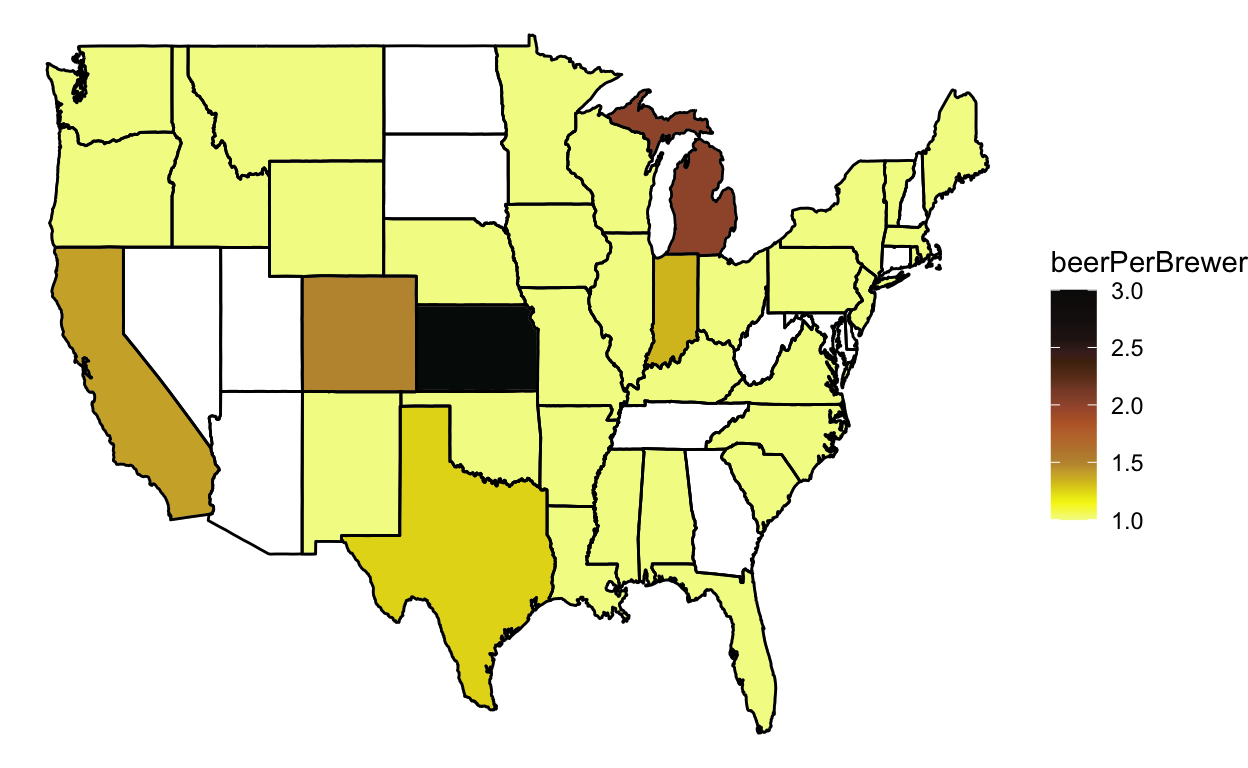What’s up with some states having no stouts?!

The controversial IPA

``````byStateI <- ipa %>%
group_by(state) %>%
summarise(numBrewer = length(unique(brewery_id)), count = n(), mabv = mean(abv, na.rm = T))

all_state <- inner_join(state, byStateI, by = c("abb" = "state"))

ggplot(data = state, mapping = aes(x = long, y = lat, group = group)) +
geom_polygon(data = all_state, aes(fill = numBrewer), color = "grey") +
labs(fill = "numBrewer") +
theme_void() +
geom_path(data = state, aes(x = long, y = lat, group = group), color = "black")
``````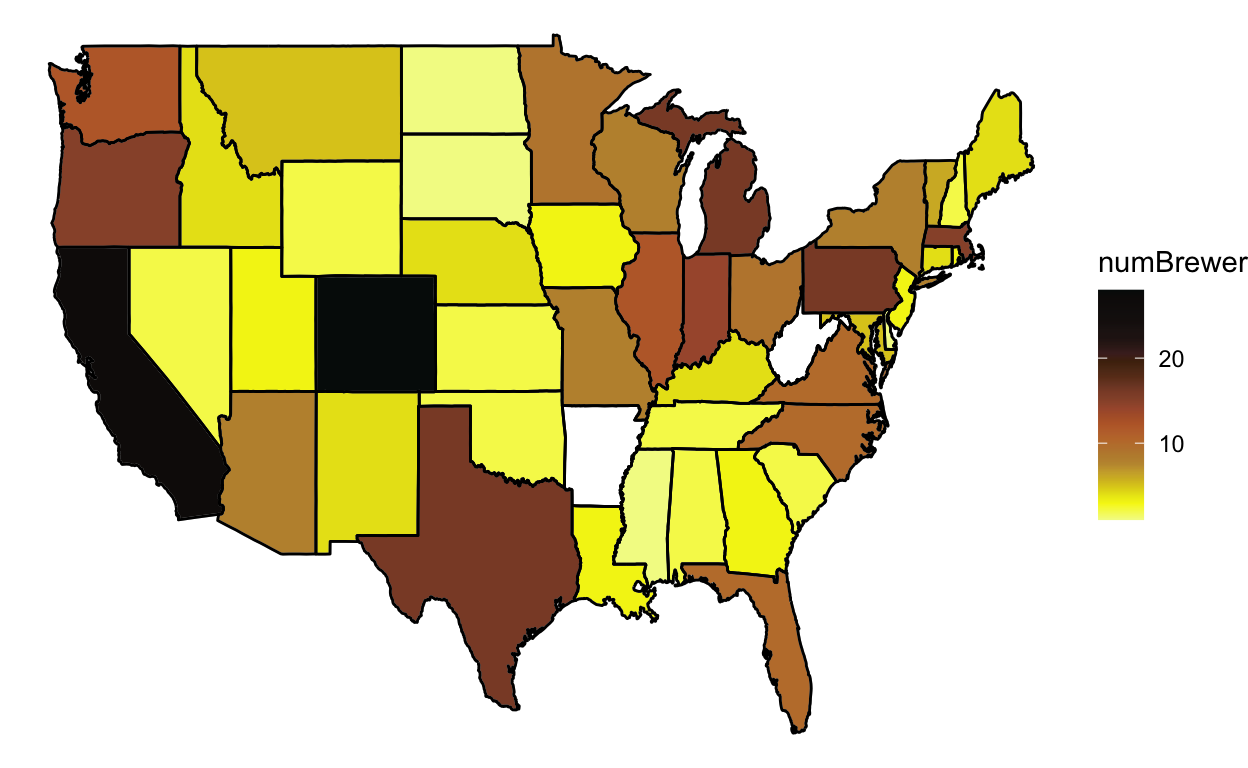``````ggplot(data = state, mapping = aes(x = long, y = lat, group = group)) +
geom_polygon(data = all_state, aes(fill = count / numBrewer), color = "grey") +
labs(fill = "beerPerBrewer") +
theme_void() +
geom_path(data = state, aes(x = long, y = lat, group = group), color = "black")
``````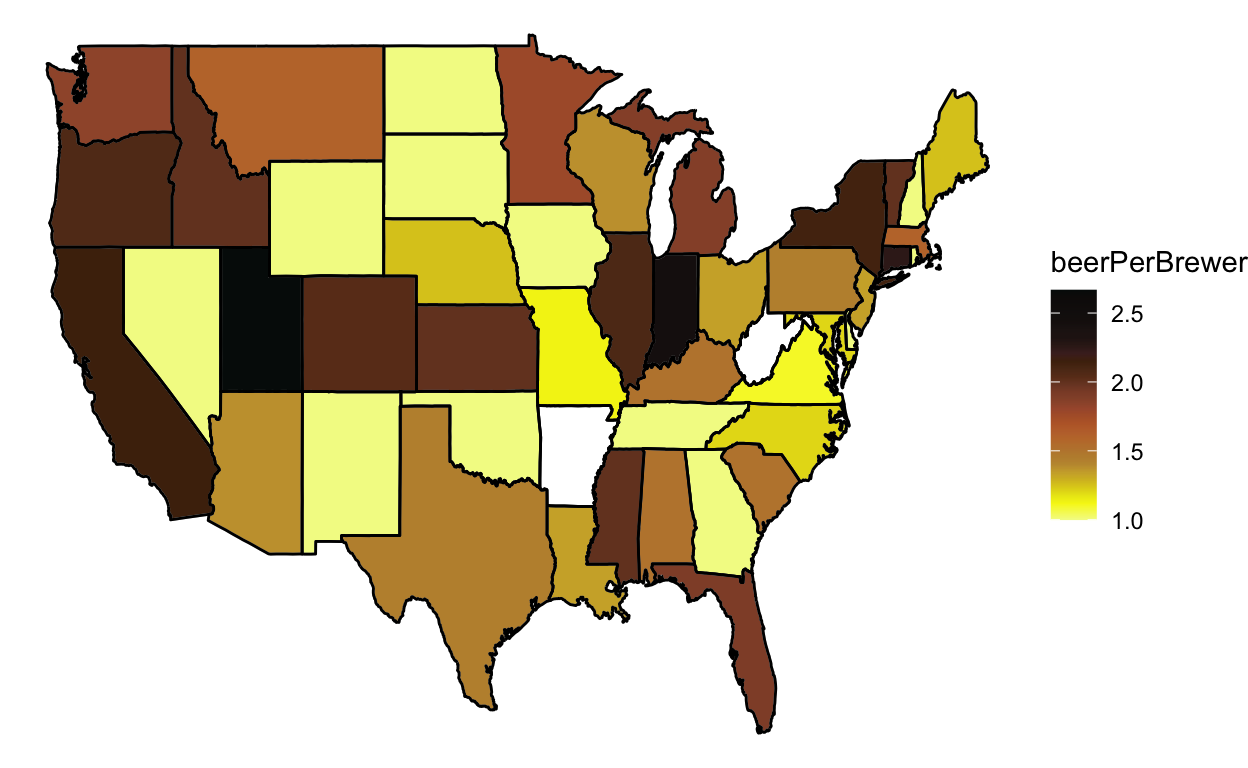``````## what's up with Utah?
ut <- beer[which(beer\$state == "UT"), ]
ut[str_detect(ut\$style, "IPA"), ] ## double counting
``````
``````# A tibble: 8 x 12
count.x   abv   ibu    id name.x style brewery_id ounces count.y
<dbl> <dbl> <dbl> <dbl> <chr>  <chr>      <dbl>  <dbl>   <dbl>
1    1382 0.04     NA   644 Johnn… Amer…        399     16     400
2    2254 0.04     42  1925 Trade… Amer…        159     12     160
3    2255 0.073    83  1723 Hop N… Amer…        159     12     160
4    2258 0.073    82  1089 Hop N… Amer…        159     12     160
5    2300 0.09     75  1825 Squat… Amer…        302     12     303
6    2302 0.06     NA  1823 Wasat… Amer…        302     12     303
7    2303 0.06     NA  1682 Wasat… Amer…        302     12     303
8    2305 0.09     75  1680 Squat… Amer…        302     12     303
# … with 3 more variables: name.y <chr>, city <chr>, state <chr>``````

West Virginia and Arkansas are not into IPAs.

### Variation in ABV

Which styles have the most variation in alcohol content (of the top 20 most prevalent styles) given their average value?

``````beer %>%
group_by(style) %>%
summarise(count = n(), coeffVarabv = mean(abv, na.rm = T) / sd(abv, na.rm = T)) %>%
arrange(desc(count)) %>%
arrange(desc(coeffVarabv))
``````
``````# A tibble: 20 x 3
style                          count coeffVarabv
<chr>                          <int>       <dbl>
1 American Double / Imperial IPA   105       12.5
2 KÃ¶lsch                           42       11.4
3 American Amber / Red Lager        29       10.7
4 American Blonde Ale              108       10.0
5 MÃ¤rzen / Oktoberfest             30        9.72
6 Hefeweizen                        40        9.44
7 American Pale Ale (APA)          245        8.63
8 Cider                             37        7.58
9 American Pale Wheat Ale           97        7.55
10 American IPA                     424        7.33
11 American Stout                    39        7.17
12 German Pilsener                   36        7.01
13 American Porter                   68        6.96
14 American Amber / Red Ale         133        6.62
15 American Pale Lager               39        6.27
16 American Brown Ale                70        5.97
17 American Black Ale                36        5.49
18 Fruit / Vegetable Beer            49        5.44
19 Saison / Farmhouse Ale            52        5.41
20 Witbier                           51        4.79``````

Fancy string matching for another time: match the beer style to the colors listed here.